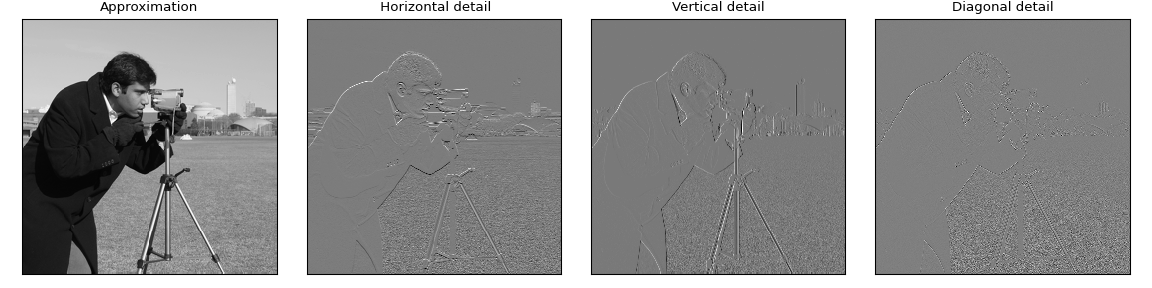# PyWavelets - Wavelet Transforms in Python¶

PyWavelets is open source wavelet transform software for Python. It combines a simple high level interface with low level C and Cython performance.

PyWavelets is very easy to use and get started with. Just install the package, open the Python interactive shell and type:

>>> import pywt
>>> cA, cD = pywt.dwt([1, 2, 3, 4], 'db1')


Voilà! Computing wavelet transforms has never been so simple :)

Here is a slightly more involved example of applying a digital wavelet transform to an image:

import numpy as np
import matplotlib.pyplot as plt

import pywt
import pywt.data

original = pywt.data.camera()

# Wavelet transform of image, and plot approximation and details
titles = ['Approximation', ' Horizontal detail',
'Vertical detail', 'Diagonal detail']
coeffs2 = pywt.dwt2(original, 'bior1.3')
LL, (LH, HL, HH) = coeffs2
fig = plt.figure(figsize=(12, 3))
for i, a in enumerate([LL, LH, HL, HH]):
ax = fig.add_subplot(1, 4, i + 1)
ax.imshow(a, interpolation="nearest", cmap=plt.cm.gray)
ax.set_title(titles[i], fontsize=10)
ax.set_xticks([])
ax.set_yticks([])

fig.tight_layout()
plt.show()## Main features¶

The main features of PyWavelets are:

• 1D, 2D and nD Forward and Inverse Discrete Wavelet Transform (DWT and IDWT)
• 1D, 2D and nD Multilevel DWT and IDWT
• 1D, 2D and nD Stationary Wavelet Transform (Undecimated Wavelet Transform)
• 1D and 2D Wavelet Packet decomposition and reconstruction
• 1D Continuous Wavelet Transform
• Computing Approximations of wavelet and scaling functions
• Over 100 built-in wavelet filters and support for custom wavelets
• Single and double precision calculations
• Real and complex calculations
• Results compatible with Matlab Wavelet Toolbox (TM)

## Getting help¶

Use GitHub Issues, StackOverflow, or the PyWavelets discussions group to post your comments or questions.